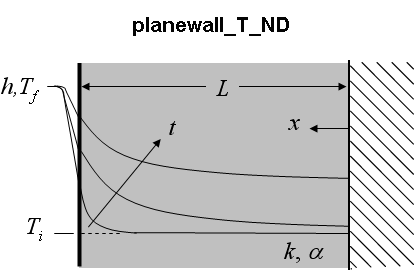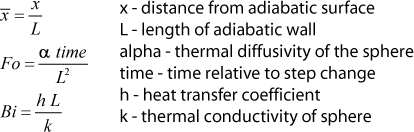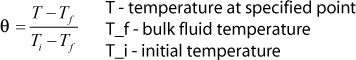Contents - Index

Planewall_T_NDThe function planewall_T_ND(x_bar, Fo, Bi) returns the non-dimensional temperature within a bounded plane wall with one edge exposed to convection (x=L) and the other adiabatic _x=0). The supplied values, x_bar, Fo, Bi represent the dimensionless position, Fourier number and Biot number respectively.

Inputs:Output:Notes:
1.  Function planewall_ND is called by the function planewall to determine the non-dimensional temperature, theta.
2.  The solutions provided by EES are the 1st 20 terms of the infinite series solution, as explained in section 3.5.2 of Nellis and Klein.
3.  The result for Fo<0.001 is determine by interpolation of the solution at Fo=0.001 and the known value (the initial condition) at Fo = 0.

Example:
x_bar=0.7
Fo=0.6
Bi=1.5
THETA=planewall_T_ND(x_bar, Fo, Bi)

{Solution: THETA=0.4946}

Transient Conduction Index